## Thermodynamic and Transport Properties of Carbon Dioxide

The site provides a feature rich, professional quality CO2 Tables web application for calculation of thermodynamic and transport properties of carbon dioxide. The CO2 Calculator Web application calculates 30 thermodynamic and transport properties of carbon dioxide. The following input variables can be used in calculations.

Input variables that can be used are:

• Pressure
• Temperature
• Enthalpy
• Entropy
• Volume
• Internal energy
• Quality (vapor mass fraction)

For thermodynamic properties the application uses new industrial formulation for carbon dioxide, formulated in Helmholtz energy. It is intended to be used for advanced technical applications. Equation of state was presented in:

• "Equations of State for Technical Applications" by R. Span and W. Wagner, International Journal of Thermophysics, Vol.24, No.1, January 2003

For transport properties the library uses equations presented in:

• "The Viscosity of Carbon Dioxide" by A. Fenghour, W. A. Wakeham and V. Vesovic
• "A Reference Multiparameter Thermal Conductivity Equation for Carbon Dioxide with an Optimized Functional Form" by G. Scalabrin, P. Marchi, and F. Finezzo and R. Span

## Thermodynamic properties

##### Range of validity

The entire set of equations used by the CO2 Calculator Web application for calculation of thermodynamic properties, is defined by the following temperature and pressure range:

• 216.6 K <= T <= 1024 K
• 0 <= p <= 100 MPa

## Transport properties

##### Range of validity
###### Viscosity of Carbon Dioxide

Equations for dynamic and kinematic viscosity are valid in the range:

• 216.6 K <= T <= 1000 K      0 <= p <= 100 MPa
• 1000 K <= T <= 1024 K      0 <= p <= 30 MPa

###### Thermal Conductivity of Carbon Dioxide

Equations for thermal conductivity and thermal diffusivity are valid in the range:

• 216.6 K <= T <= 1000 K
• 0 <= p <= 100 MPa

###### Prandtl Number

Prandtl number is dependent of dynamic viscosity and thermal conductivity, so it has range of validity according to these properties.

## Reference State

For carbon dioxide, the enthalpy and the entropy of the ideal gas is set to zero at:

• T0 = 298.15 K
• p0 = 0.101325 MPa

## Input variables

To calculate properties, CO2 Tables Web application allows up to 11 different combinations of input variables in SI or English units:

•  1 f (pressure, temperature)
•  2 f (pressure, enthalpy)
•  3 f (pressure, entropy)
•  4 f (pressure, specific volume)
•  5 f (pressure, internal energy)
•  6 f (temperature, enthalpy)
•  7 f (temperature, entropy)
•  8 f (temperature, specific volume)
•  9 f (temperature, internal energy)
• 10f (pressure, quality)
• 11f (temperature, quality)

Range of validity for listed functions:

• Functions 1-9 are valid in all regions defined by the application
• Functions 10 and 11 are valid in saturation region only

For input pressure, always enter absolute pressure and not gauge pressure.

## Available Properties

The following 30 thermodynamic and transport properties can be calculated with the CO2 Calculator:

•  1 Pressure, p
•  2 Temperature, t
•  3 Density, d
•  4 Specific volume, v
•  5 Specific enthalpy, h
•  6 Specific entropy, s
•  7 Specific exergy, ex
•  8 Specific internal energy, u
•  9 Specific isobaric heat capacity, cp
• 10Specific isochoric heat capacity, cv
• 11Speed of sound, w
• 12Isentropic exponent, kapa
• 13Specific Helmholtz free energy, H
• 14Specific Gibbs free energy, G
• 15Compressibility factor, Z
• 16Quality [vapor mass fraction], x
• 17Isobaric volume expansion coefficient, alpha
• 18Isothermal compressibility, Kt
• 19Partial derivative (dV/dT)p, dvdt
• 20Partial derivative (dV/dP)T, dvdp
• 21>Partial derivative (dP/dT)v, dpdt
• 22Partial derivative (dP/dV)T, dpdv
• 23Isothermal Joule-Thomson coefficient, iJTC
• 24Joule-Thomson coefficient, JTC
• 25Melting pressure, pm
• 26Dynamic viscosity, dv
• 27Kinematic viscosity, kv
• 28Thermal conductivity, tc
• 29Thermal diffusivity, td
• 30Prandtl number, Pr

## Open new file

To open a new file, do the following:

• Choose File, Open menu item

Alternatively, press the "New" toolbar button.

This will clear all currently displayed data on the forms.

## Save data to Excel

To save calculated properties to your local computer, use the following steps:

• Alternatively, choose File, Save to Excel as CSV Unicode menu item. The File Download dialog appears
• Choose Save to save the document to your computer

The CSV Unicode format will save properly some special characters contained in the definition of units. Since CSV is just a text file you can open a saved file with any text editor, for example Notepad.

## Select Units

On the "Setup Pane" in the "Units" group box, set units as required. SI and English units are available.

## Ambient Condition

On the "Setup Pane" In the "Ambient" group box, set a reference temperature of the environment. After entering a temperature press the "Update" button. The reference temperature will be used for the calculation of exergy.

## Calculate Properties

At the top of the CO2 Table Calculator Page the first two tabs enable you to select between "General properties" and "Saturation properties". On the "General Properties" tab you will be able to calculate properties in all regions, as defined by the application, including saturation properties. For example, if you enter input data that define a point inside the saturation region, properties will be calculated and displayed in the same way as properties for all other regions. On the other hand, on the second tab you will be able to calculate properties only in saturation region.

##### Calculation procedure for all regions

In order to calculate properties valid in all regions, select the first tab "General properties", and follow next steps:

• Choose units, SI or English
• Select one of the 9 different functions based on available input variables
• Enter data for the first parameter
• Enter data for the second parameter
• Press "Calculate" button to perform calculation

Detailed result of a calculation is displayed in the main table, located in the lower part of the input form, and also 12 main properties are displayed in the "Output pane". Input arguments used in a calculation are displayed in red.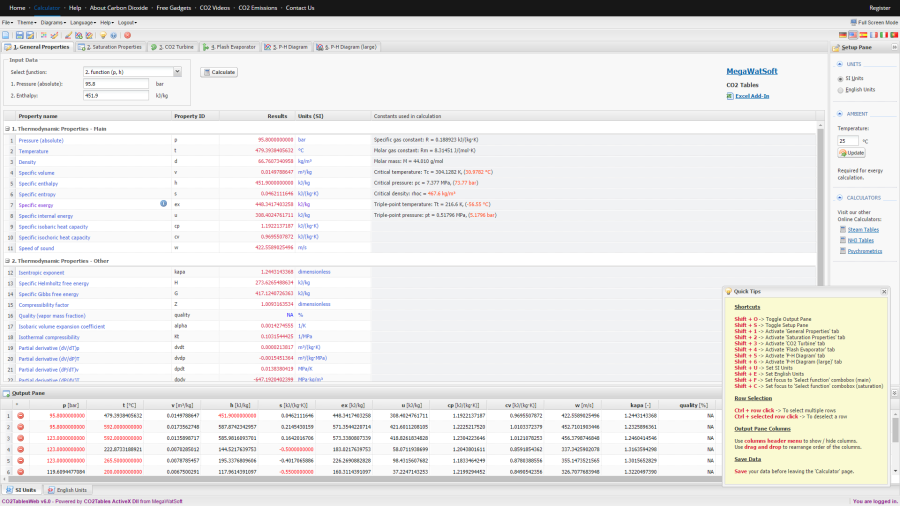Fig.1 Calculation of General properties of carbon dioxide

##### Calculation procedure for the saturation region

In order to calculate properties in the saturation region, select the second tab "Saturation properties" and follow next steps:

• Choose units, SI or English
• Select one of the 2 different functions based on available input variables
• Enter data for the first parameter
• Enter data for the second parameter
• Press "Calculate" button to perform calculation

Detailed result of a calculation is displayed in the main table, located in the lower part of the input form, and also 12 main properties are displayed in the "Output pane". Input arguments used in a calculation are displayed in red.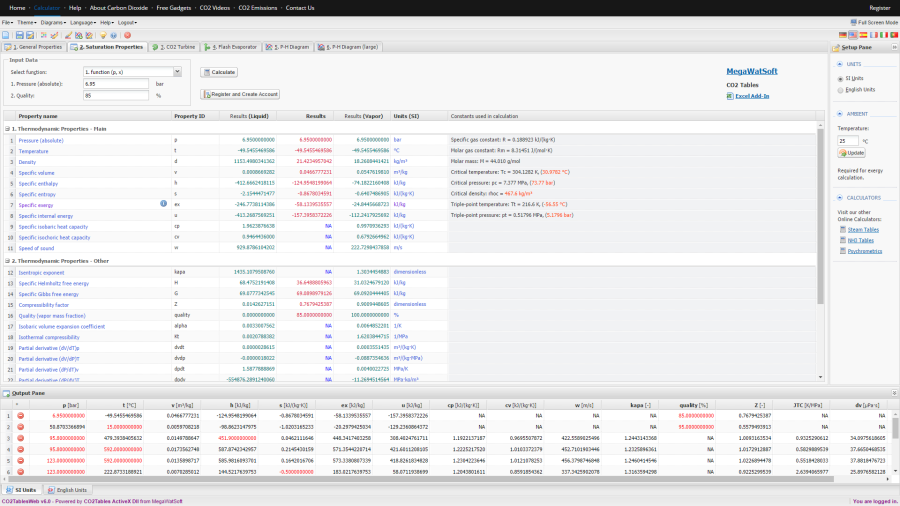Fig.1 Calculation of Saturation properties of carbon dioxide

In the Output Pane results are always inserted at the top, so you don't have to scroll to see the latest result.

## CO2 turbine calculations

##### Calculate steam consumption of a CO2 turbine

To calculate steam consumption of a carbon dioxide turbine, follow these steps:

• Select "CO2 Turbine" tab
• In the "Select Calculator" group box select "Turbine steam consumption calculator" radio button
• Enter required input data in the "Input Data" group box
• Press "Calculate" button

Results of the calculation will be displayed in the first empty "results" column in the main pane.

##### Calculate CO2 efficiency

To calculate carbon dioxide turbine efficiency, follow these steps:

• Select "CO2 Turbine" tab
• In the "Select Calculator" group box select "Turbine efficiency calculator" radio button
• Enter required input data in the "Input Data" group box
• Press "Calculate" button

Results of the calculation will be displayed in the first empty "results" column in the main pane.

##### Calculate CO2 turbine power

To calculate carbon dioxide turbine power, follow these steps:

• Select "CO2 Turbine" tab
• In the "Select Calculator" group box select "Turbine power calculator" radio button
• Enter required input data in the "Input Data" group box
• Press "Calculate" button

Results of the calculation will be displayed in the first empty "results" column in the main pane.

Before making the fourth calculation, press the "Clear results" button to clear results from the 3 available result columns in the main pane.

For each CO2 turbine calculation, calculated inlet and exhaust conditions of the turbine will be displayed in the "Output pane" as well. To distinguish turbine results from other data, these rows will be displayed in green.

## Flash evaporator calculations

##### Calculate flash evaporation of condensate at saturation

To calculate flash evaporation of condensate at saturation, follow these steps:

• Select "Flash Evaporation" tab
• In the "Condensate" group box select "Condensate at saturation" radio button
• Enter required input data in the "Input Data" group box
• Press "Calculate" button

Results of the calculation will be displayed in the first empty row in the main pane.

##### Calculate flash evaporation of subcooled condensate

To calculate flash evaporation of subcooled condensate, follow these steps:

• Select "Flash Evaporation" tab
• In the "Condensate" group box select "Subcooled condensate" radio button
• Enter required input data in the "Input Data" group box
• Press "Calculate" button

Results of the calculation will be displayed in the first empty row in the main pane.

For each flash evaporator calculation, calculated inlet and outlet conditions will be displayed in the "Output pane" as well. To distinguish flash evaporation results from other data, inlet properties will be displayed in blue and outlet properties in red.

To display point(s) on CO2 Mollier diagrams use the following steps:

• Calculate a few points you want to display
• Click on the "Diagrams, Add / Edit Points" menu item, the following dialog box should open
• If you work with SI units select the SI Units Tab radio button" otherwise select English units radio button
• In the "Displayed Point ID's" edit box type ID's of the points you want to display from the ID's column in the Output Pane, for example to display the first 3 points type: 1,2,3
• In the "Displayed Point labels" edit box type the labels (of your choice) of the respective points, for example: a,b,c
• The number of points and the number of labels should match
• Press the "Save" button and points will be displayed on all diagrams (Fig.5)

OPTIONALY - You may open "Setup UI for Points" dialog box (Fig.4), by choosing "Diagrams, Setup UI for Points" menu item and change default radius and color of the points. This UI setup will be applied to all displayed points.Fig.3 Add points to CO2 Mollier diagrams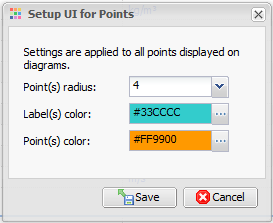Fig.4 Setup UI for points displayed on CO2 diagrams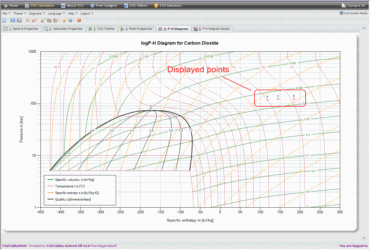Fig.5 Displayed points on CO2 P-H Diagram

Diagrams are available only in SI units.

For calculations performed in English units, points are first converted to SI units and then displayed on SI diagrams.

## Edit Point(s)

To edit point(s) already displayed on the diagrams use the following steps:

• Click on the "Diagrams, Add / Edit Points" menu item to open "Add / Edit Points" dialog box
• In the "Displayed Point ID's" edit box make required changes
• In the "Displayed Point Labels" edit box make required changes
• Press the "Save" button and modified points will be displayed on all diagrams

## Add a Line or a Curve

To display line(s) or curve(s) on the diagrams use the following steps:

• Calculate and display a few points on the diagrams (see the Add Points section)
• Click on the "Diagrams, Add a Line or a Curve" menu item to open "Add a Line or a Curve" dialog box
• In the edit box "Add labels to define a new series", add labels: a,b,c
• In the edit box "Add name of the series" type a name of a line/curve, for example: test
• In the "Set type of series" combo box select line or spline option. Spline is used to draw curves and you must provide at least 3 points to make it effective
• Press the "Save" button and lines and curves will be displayed on all diagrams (Fig.8)

OPTIONALY - You may open "Setup UI for Lines and Curves" dialog box (Fig.7), by choosing "Diagrams, Setup UI for Lines and Curves" menu item and change default width and color of the lines and curves. This UI setup will be applied to all displayed lines and curves.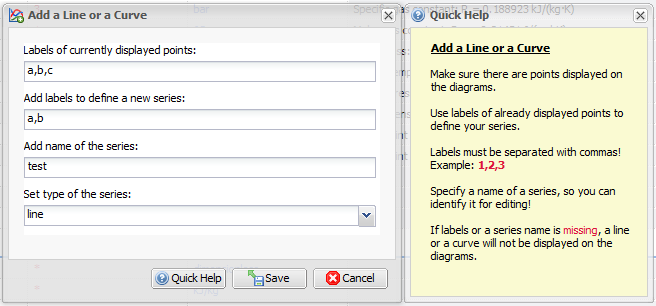Fig.6 Add a process to Mollier diagrams

Edit box "Labels of currently displayed points" will show you available points for the creation of line(s) and curve(s).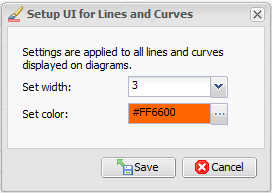Fig.7 Setup UI for lines and curves on Mollier diagrams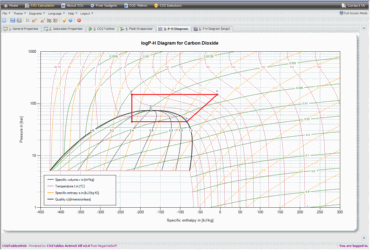Fig.8 Processes displayed on CO2 P-H diagram

## Edit a Line or a Curve

To edit a line or a curve already displayed on the CO2 diagrams use the following steps:

• Click on the "Diagrams, Edit a Line or a Curve" menu item to open "Edit a Line or a Curve" dialog box
• In the combo box "Select a series to edit" choose a line or a curve you want to modify
• Make required changes to the currently selected line or curve
• Press the "Save" button to close the dialog box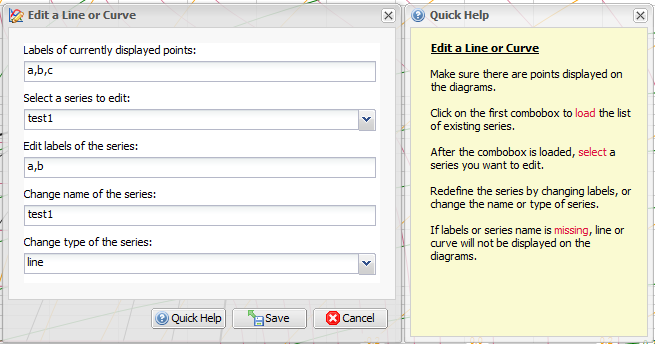Fig.9 Edit UI of processes displayed on CO2 Mollier diagrams

## Save Diagram

To save a diagram use the following steps:

• Click the right mouse button on a diagram